Function Repository Resource:

# RoundedAnnulus3D

Represent a 3D annulus with rounded edges

Contributed by: Jaroslav Kysela
 ResourceFunction["RoundedAnnulus3D"][{{x1,y1,z1},{x2,y2,z2}},{rinner,router}] represents a 3D annulus around the line from {x1,y1,z1} to {x2,y2,z2} with rounded edges with inner radius rinner and outer radius router.

## Details and Options

ResourceFunction["RoundedAnnulus3D"] can be used in Graphics3D.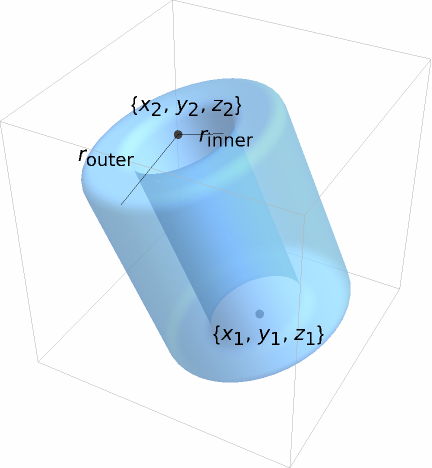Graphics rendering is affected by directives such as FaceForm, Specularity, Opacity and color.
ResourceFunction["RoundedAnnulus3D"][] is equivalent to ResourceFunction["RoundedAnnulus3D"][{{0,0,-1},{0,0,1}},{1/2,1}].
ResourceFunction["RoundedAnnulus3D"][r] is equivalent to ResourceFunction["RoundedAnnulus3D"][{{0,0,-1},{0,0,1}},{r/2,r}].
ResourceFunction["RoundedAnnulus3D"][{rinner,router}] is equivalent to ResourceFunction["RoundedAnnulus3D"][{{0,0,-1},{0,0,1}},{rinner,router}].
ResourceFunction["RoundedAnnulus3D"][{{x1,y1,z1},{x2,y2,z2}}] is equivalent to ResourceFunction["RoundedAnnulus3D"][{{x1,y1,z1},{x2,y2,z2}},{1/2,1}].
ResourceFunction["RoundedAnnulus3D"][{{x1,y1,z1},{x2,y2,z2}},r] is equivalent to ResourceFunction["RoundedAnnulus3D"][{{x1,y1,z1},{x2,y2,z2}},{r/2,r}].
The following options can be given:
The RoundingRadius setting can have the following forms:
 r use r for both the inner and the outer radius {rin,rout} separate setting for both radii
ResourceFunction["RoundedAnnulus3D"] allows 0rinnerrouter.

## Examples

### Basic Examples (3)

A unit radius and two units height 3D annulus:

 In:=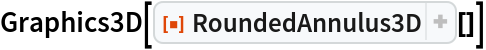Out=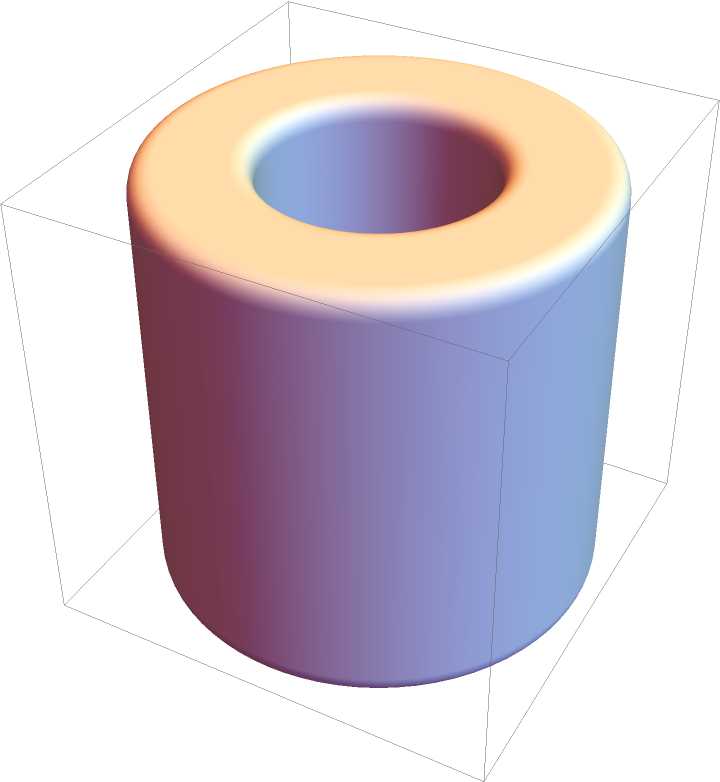A 3D annulus from the origin to {1,-1,1} with inner radius 1/2:

 In:=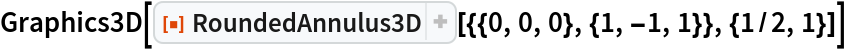Out=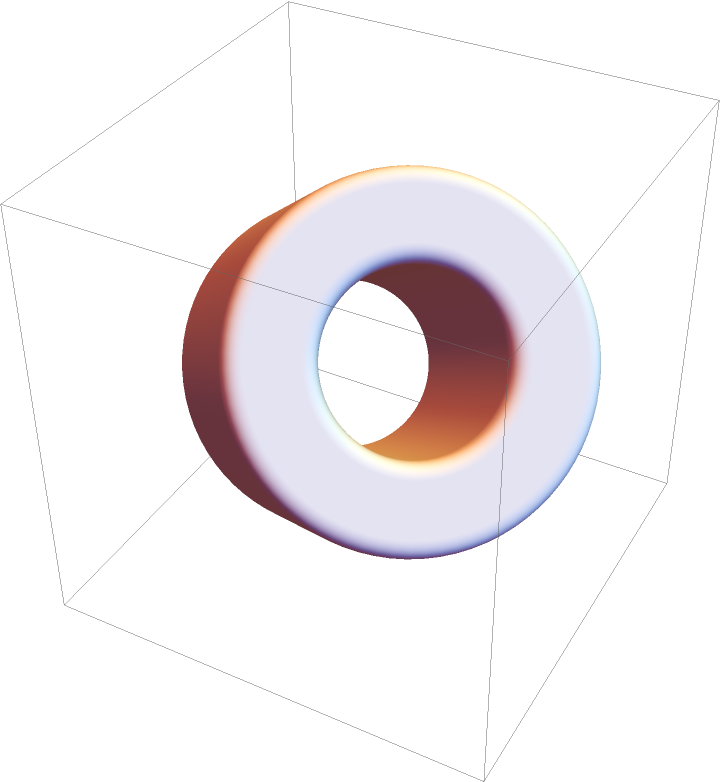Differently styled annuli:

 In:=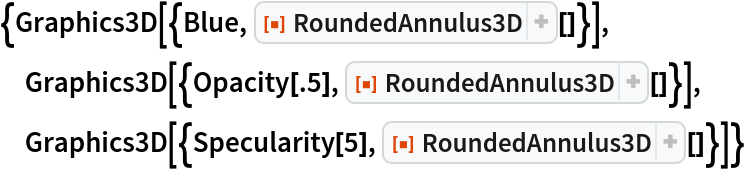Out=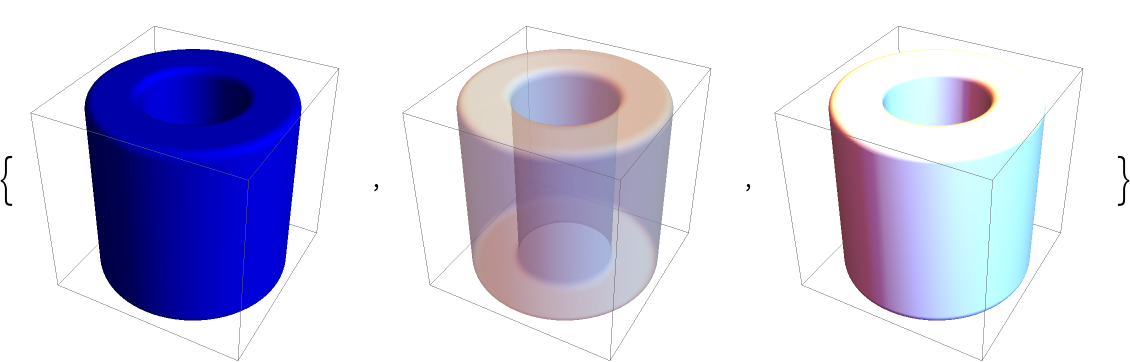### Scope (10)

#### Specification (5)

 In:=Out=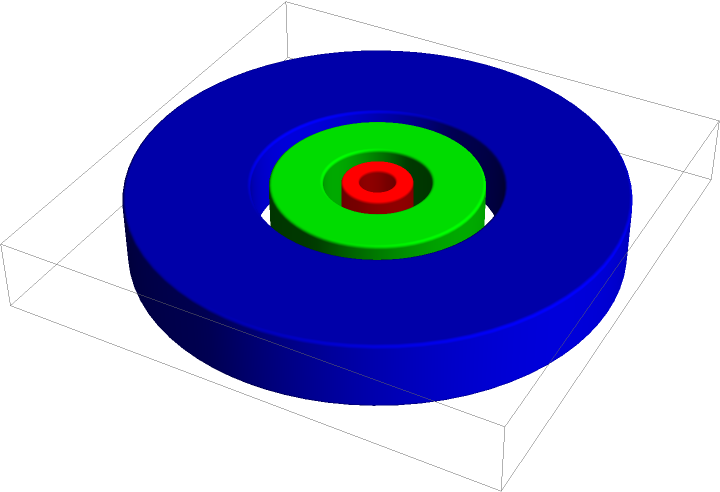Specify centers:

 In:=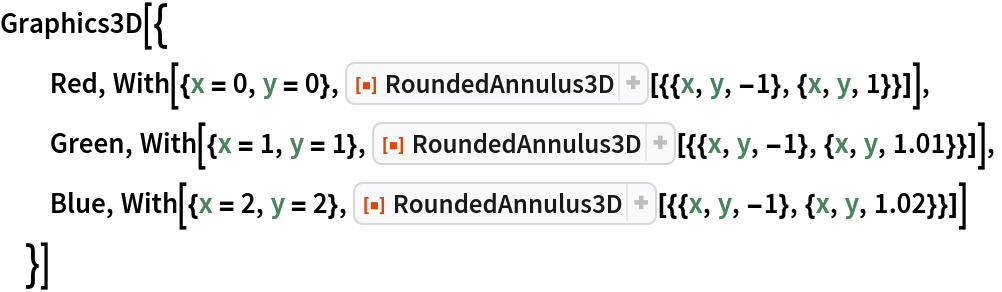Out=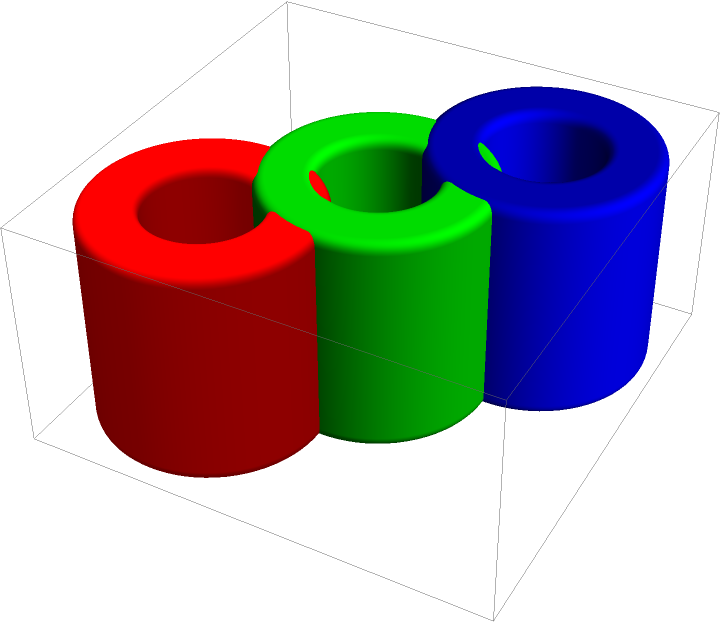Annuli with different directions:

 In:=Out=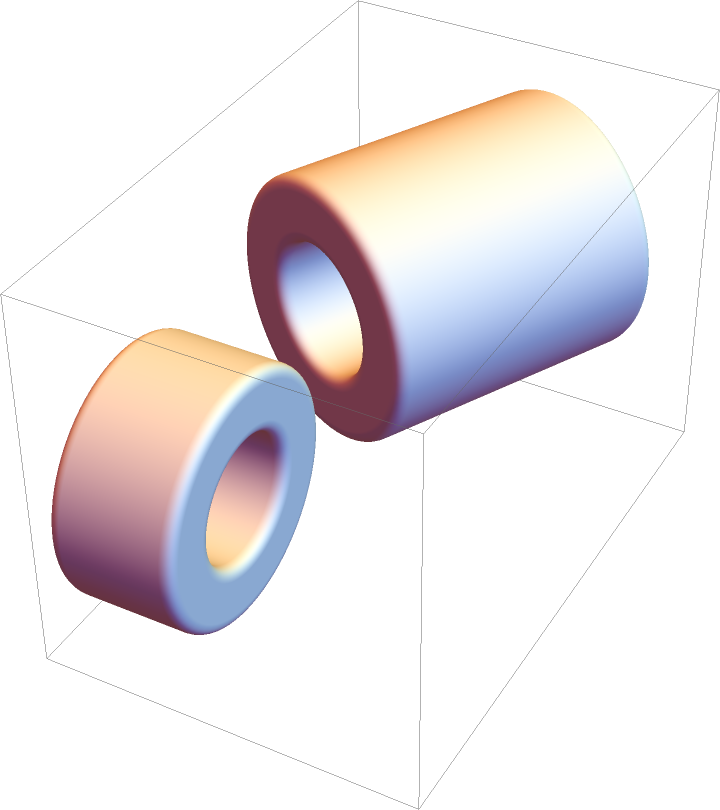Specify position, direction, as well as inner and outer radii:

 In:=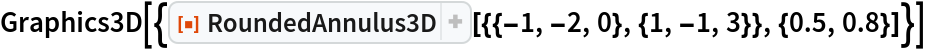Out=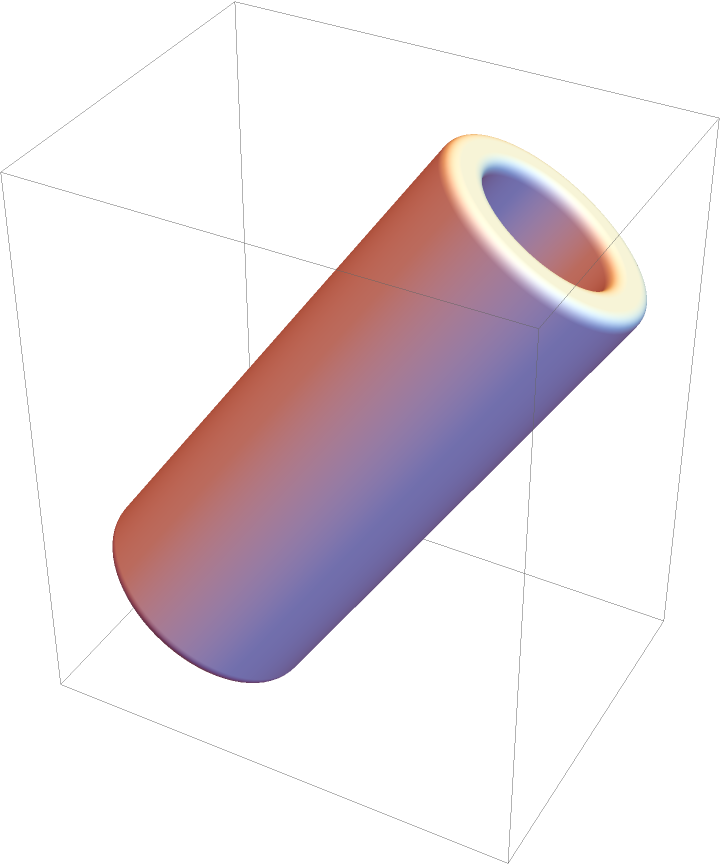Short form for a 3D annulus centered at the origin with a radius 1:

 In:=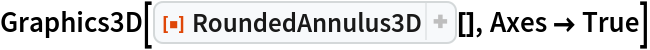Out=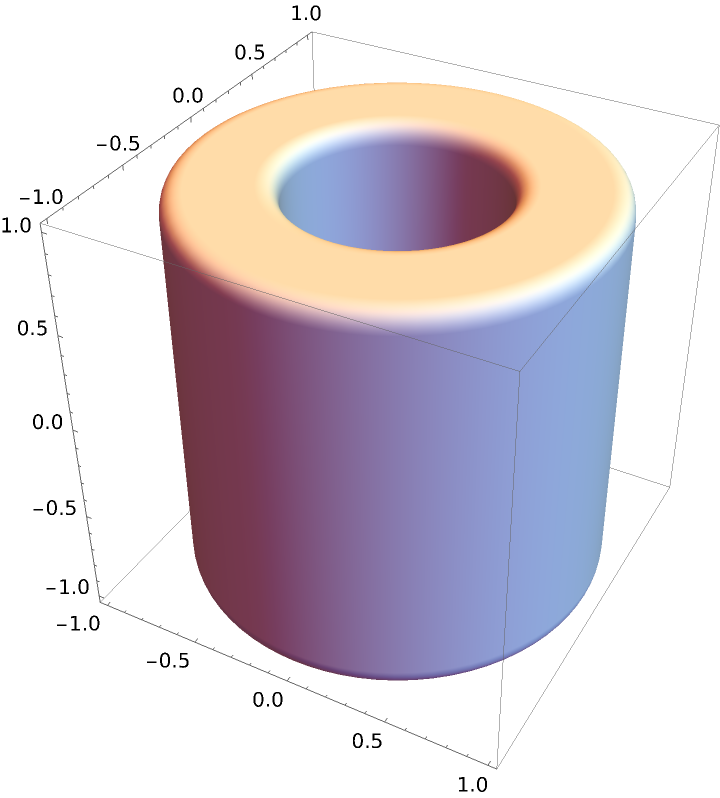#### Styling (5)

Color directives specify the face color of each annulus:

 In:=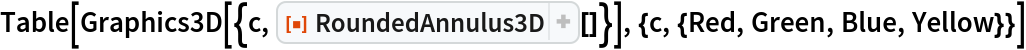Out=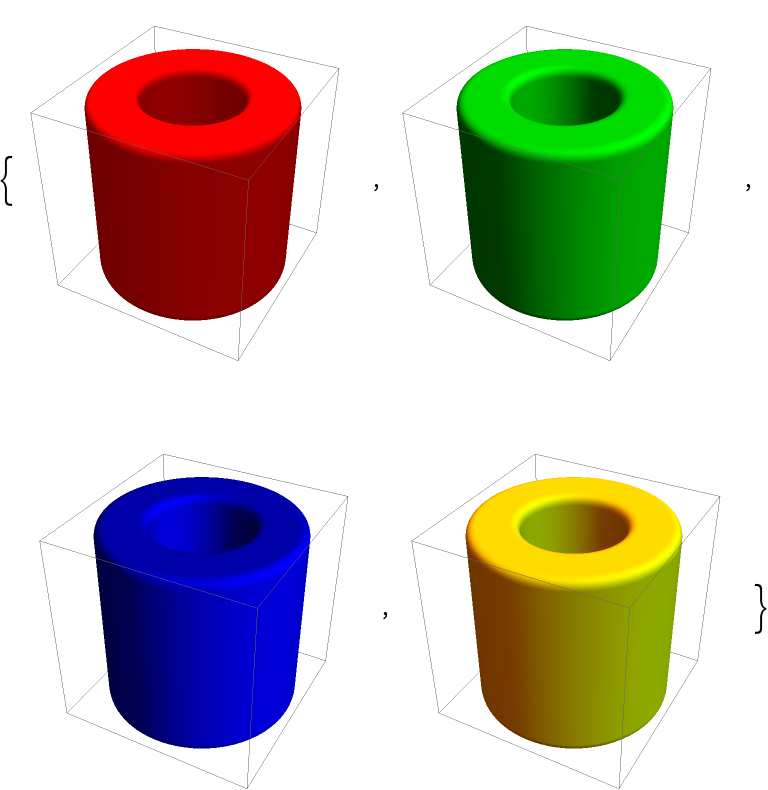FaceForm can be used to specify the styles of the interiors as well as exteriors:

 In:=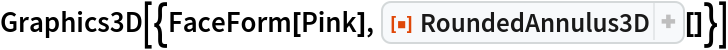Out=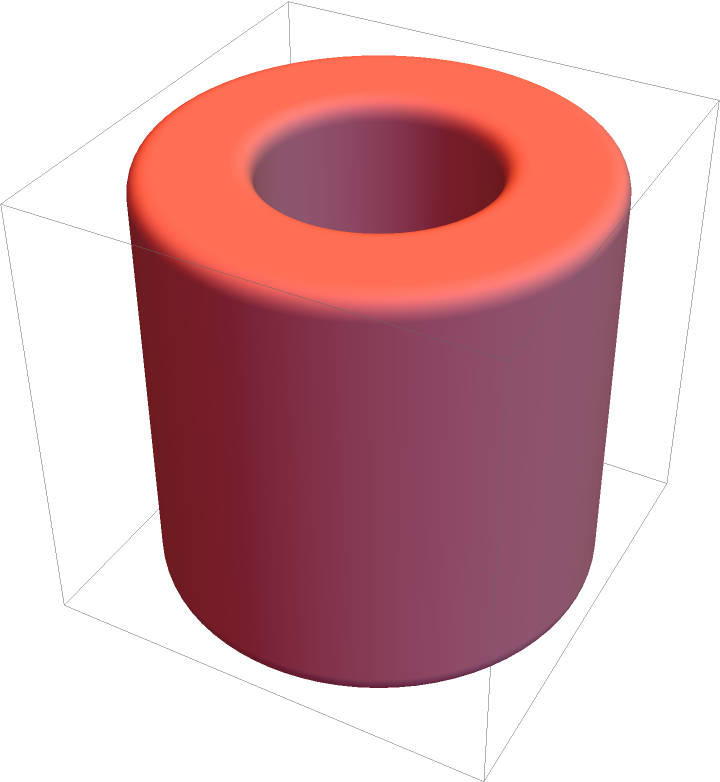Different properties can be specified for the front and back faces using FaceForm:

 In:=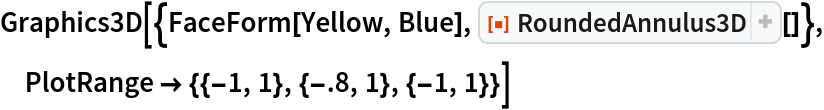Out=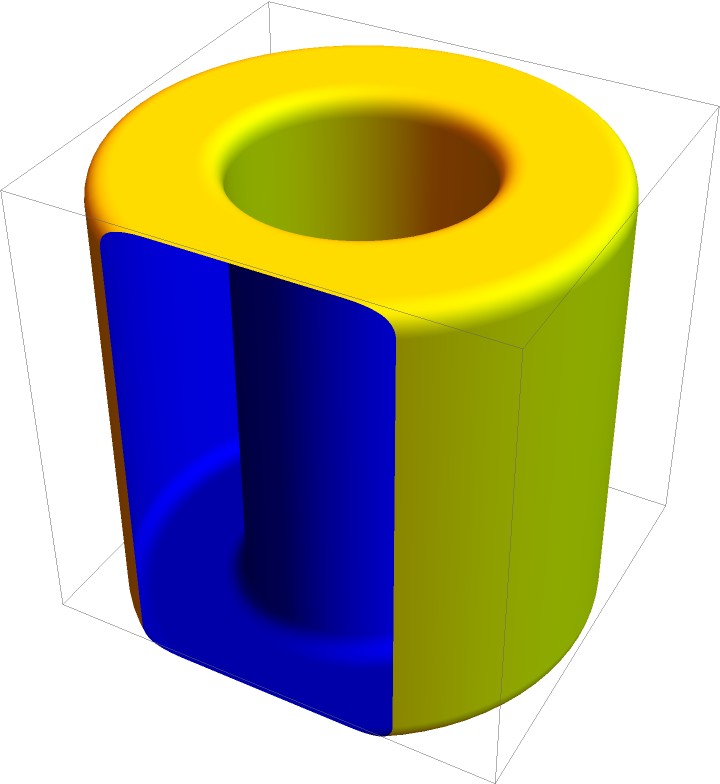Opacity specifies the face opacity:

 In:=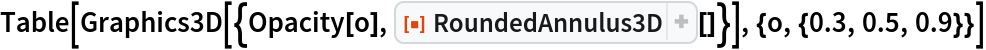Out=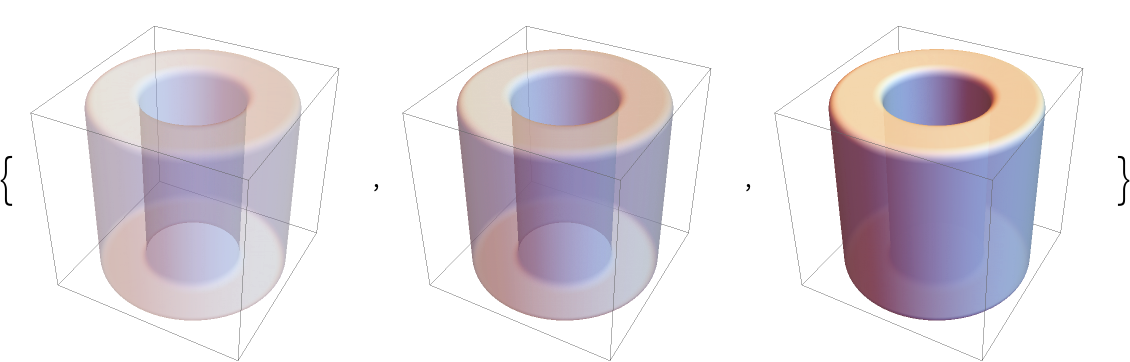Decompose the annulus into its components and apply different styling to each:

 In:=In:=Out=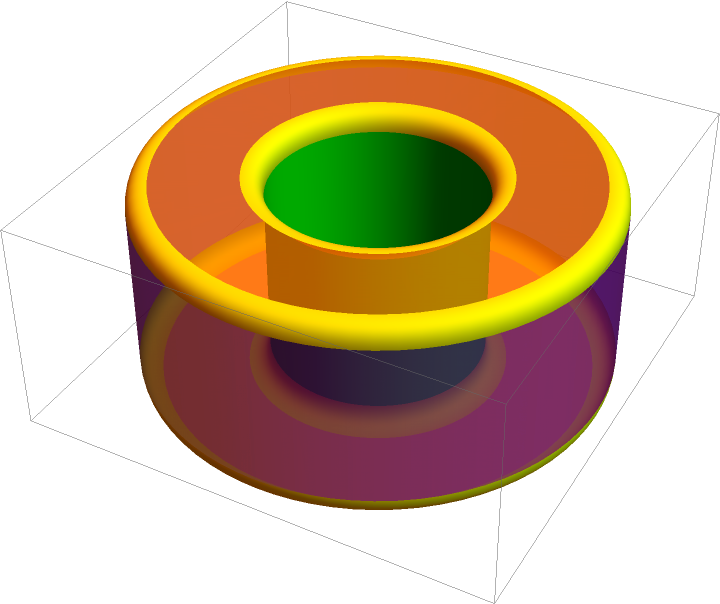### Options (2)

Adjust how rounded the edges are:

 In:=Out=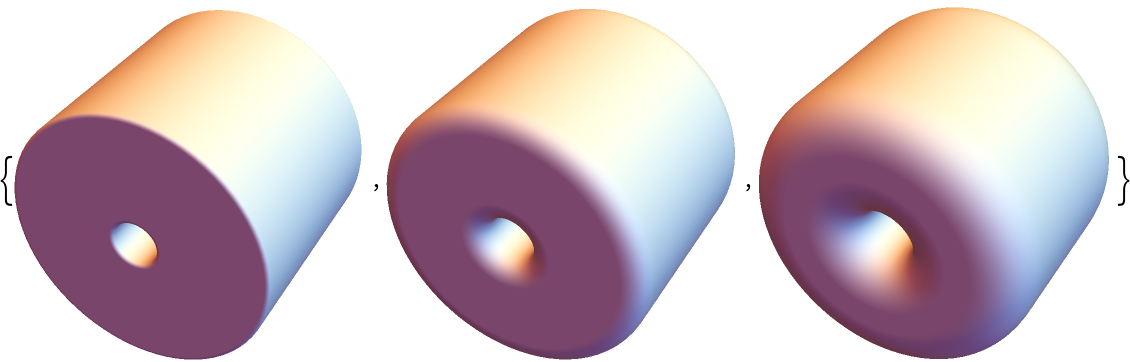Adjust the inner and outer edges independently:

 In:=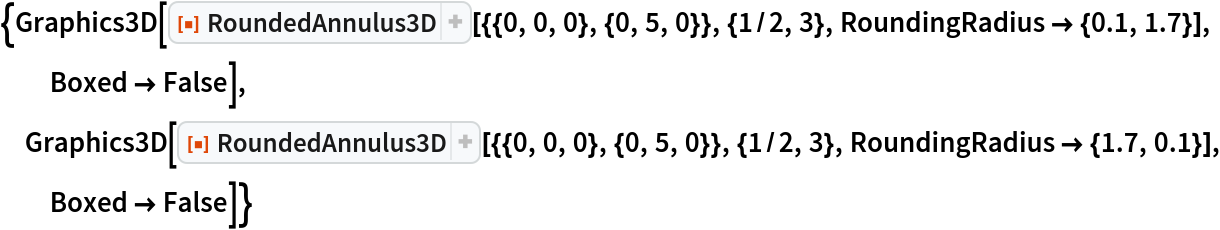Out=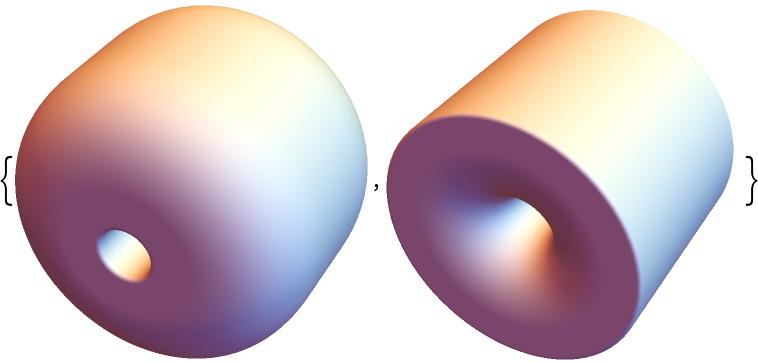### Applications (2)

Create a model of a wheel:

 In:=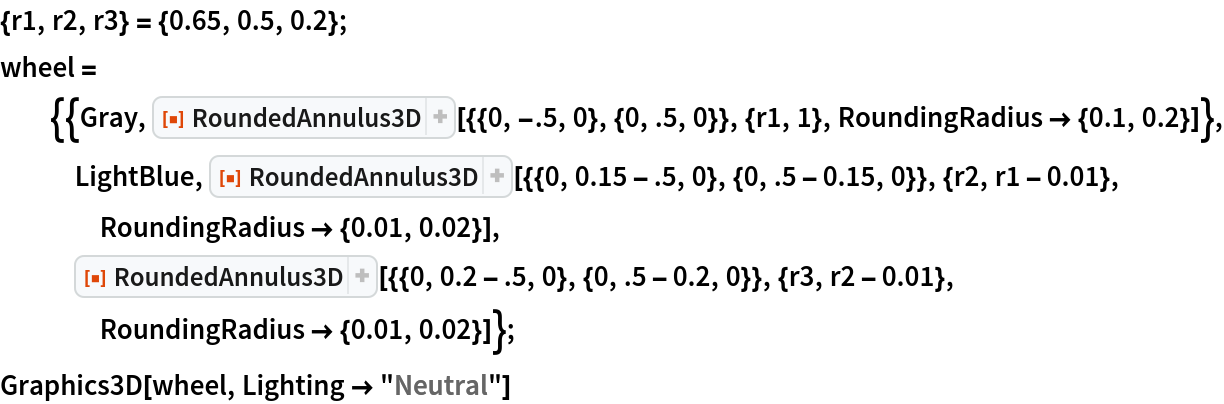Out=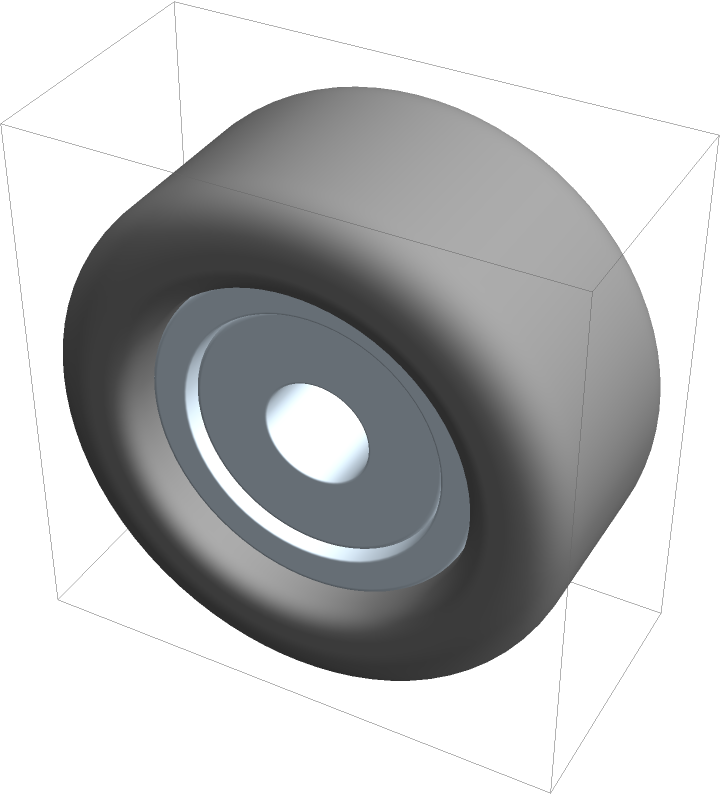Use it to create a model of a chassis:

 In:=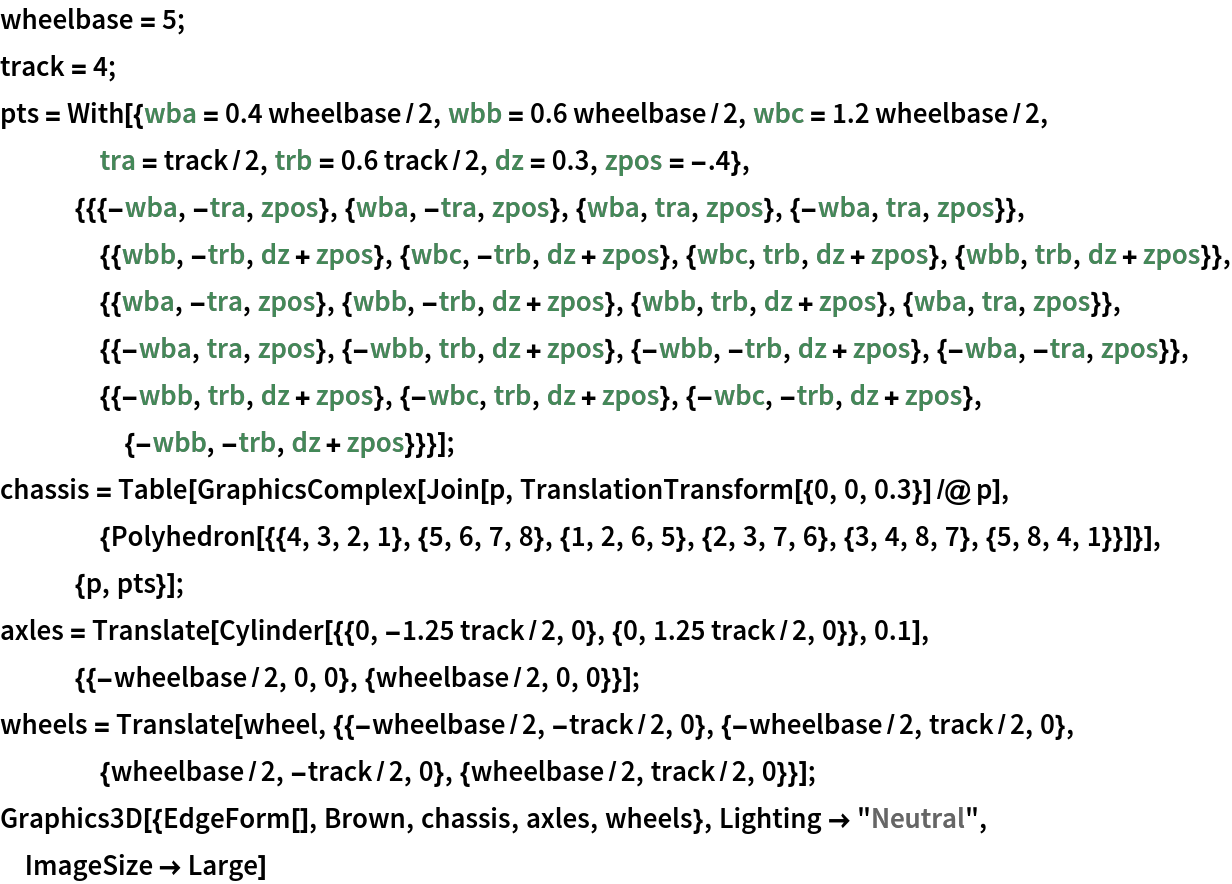Out=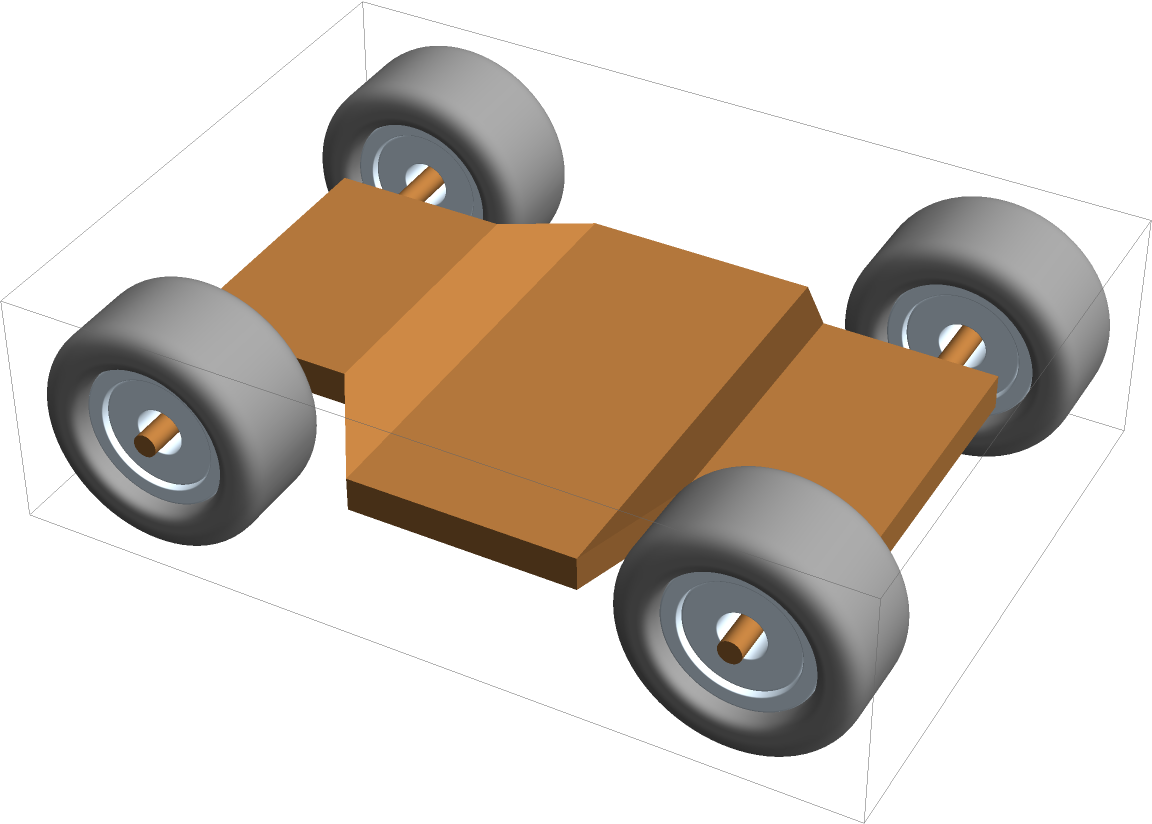### Properties and Relations (4)

RoundedAnnulus3D is a 3D generalization of Annulus in 2D:

 In:=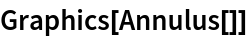Out=The resource function RoundedCylinder effectively corresponds to RoundedAnnulus3D with zero inner radius:

 In:=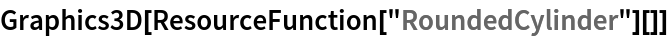Out=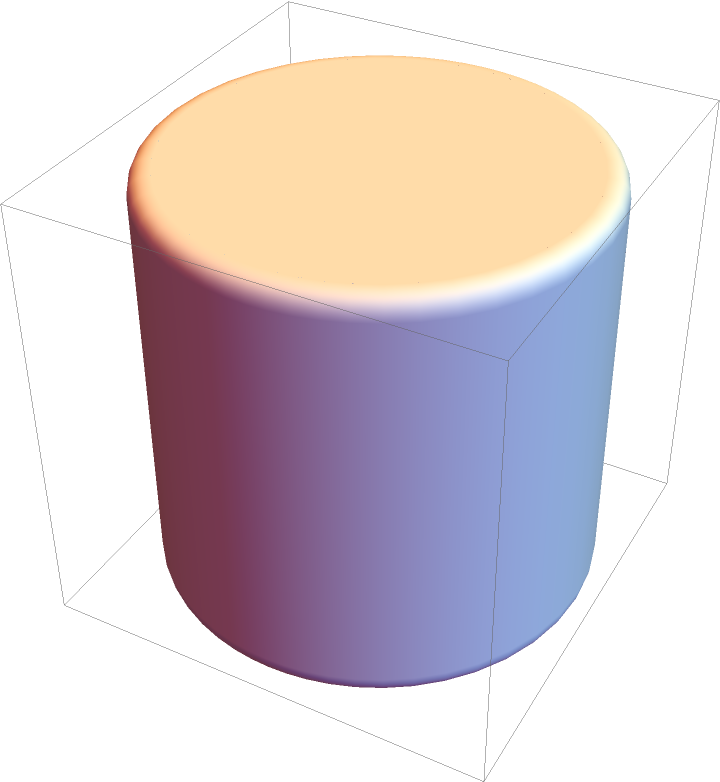PieChart3D or SectorChart3D can be used to create 3D rounded annuli:

 In:=Out=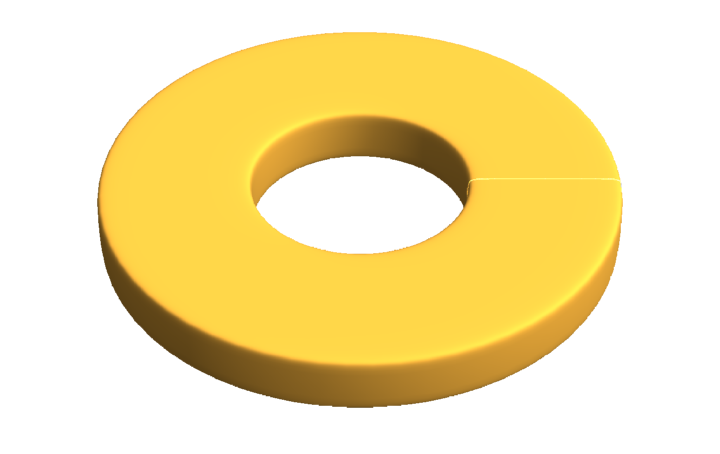Get the graphical primitive from PieChart3D:

 In:=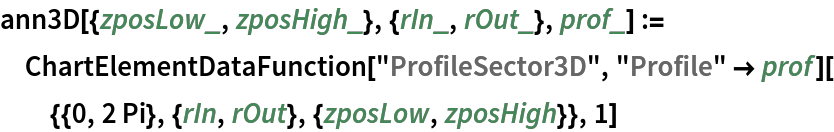In:=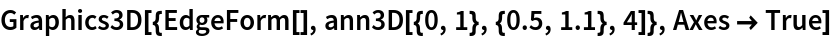Out=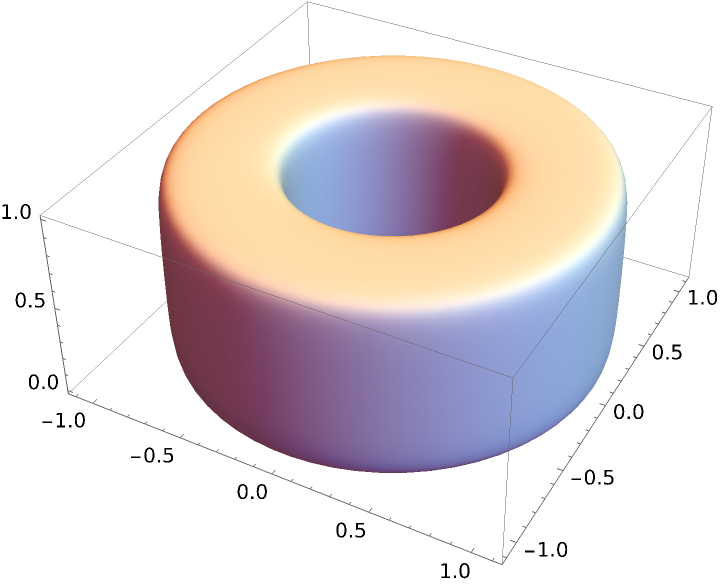Compare with RoundedAnnulus3D:

 In:=Out=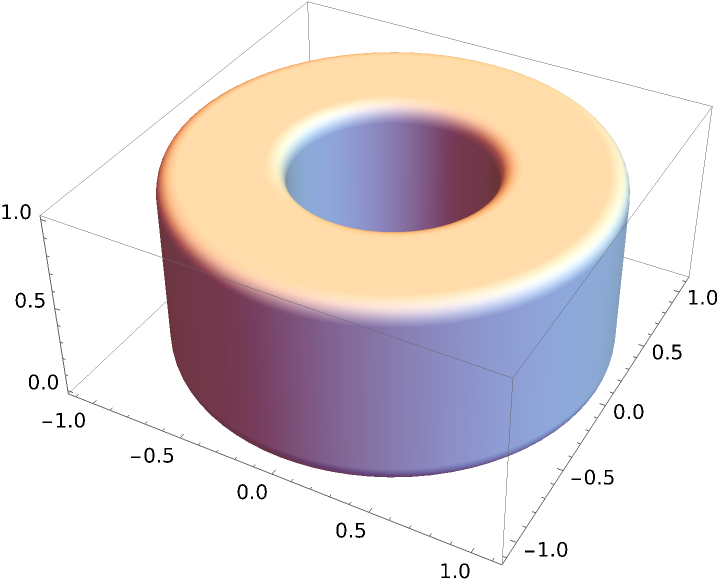Despite being seemingly the same, the two annuli have a very different internal structure:

 In:=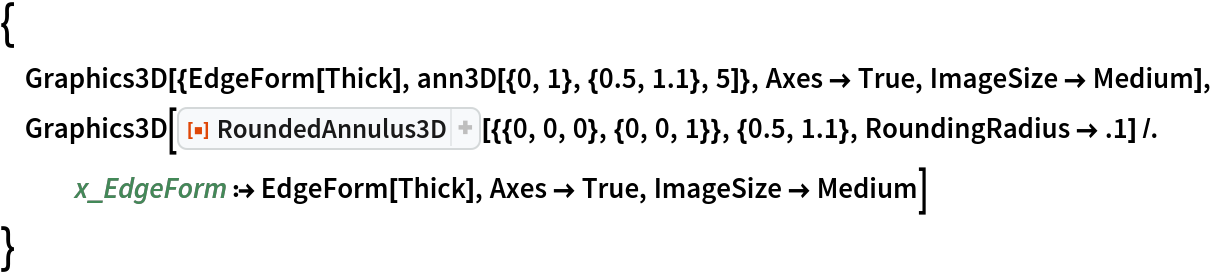Out=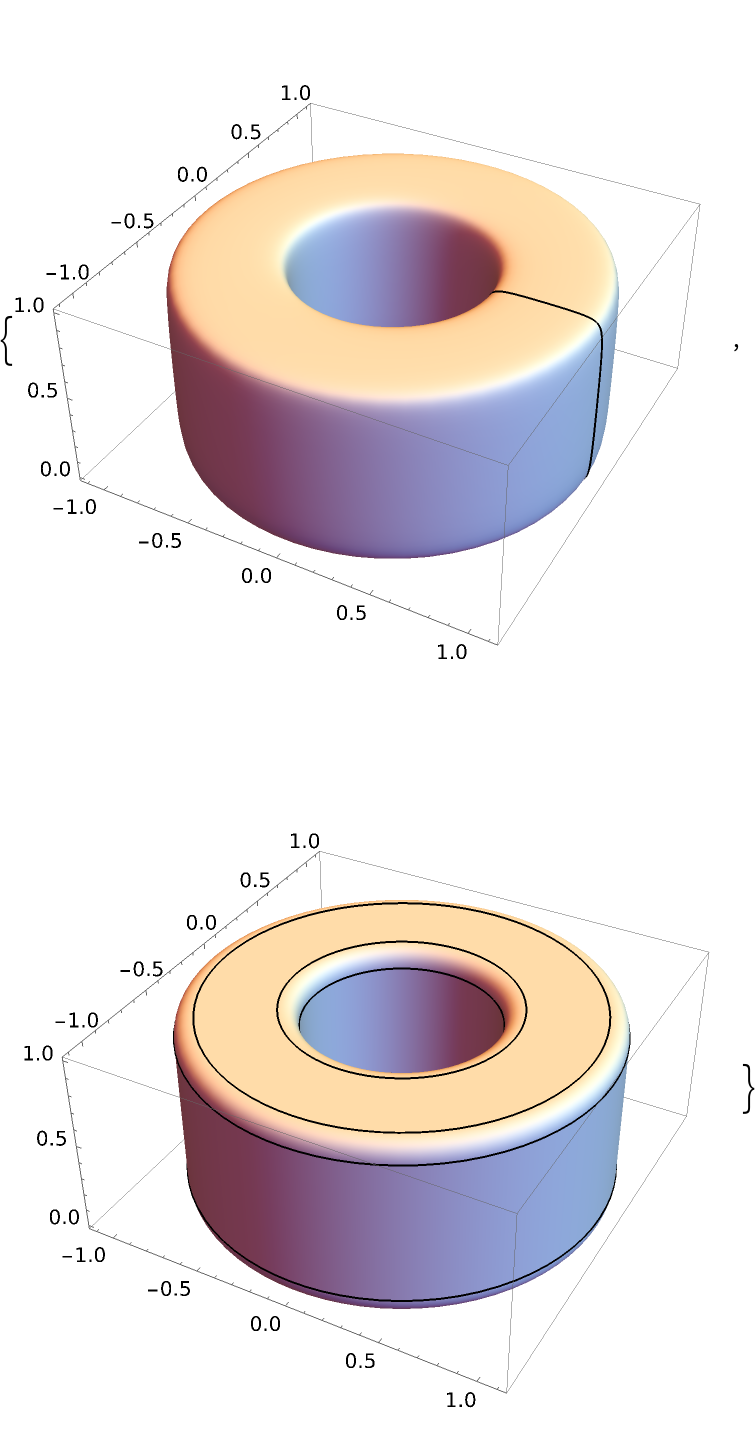### Possible Issues (5)

When the two endpoints coincide, no annulus is created:

 In:=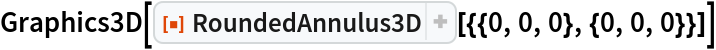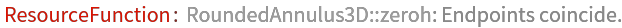Out=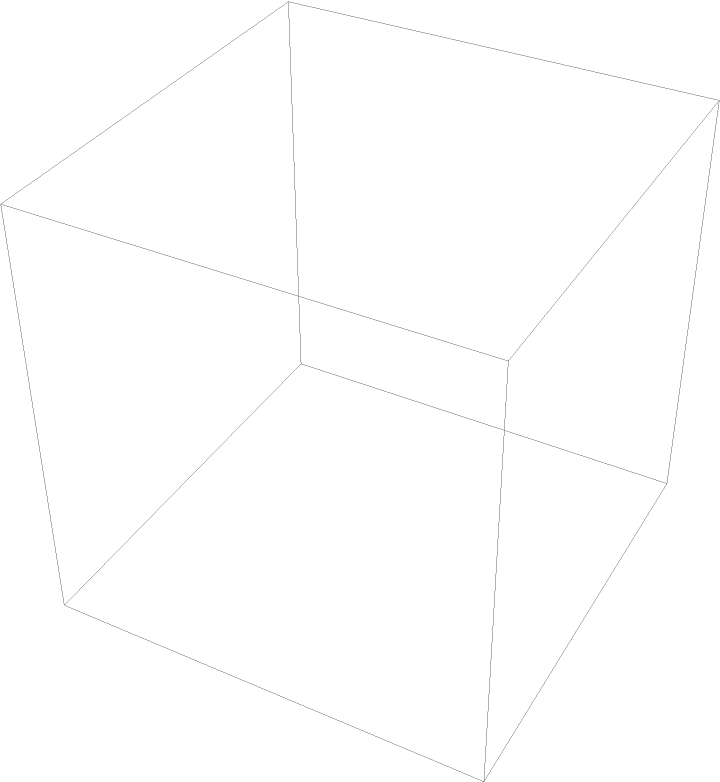Radii are assumed to be non-negative real numbers:

 In:=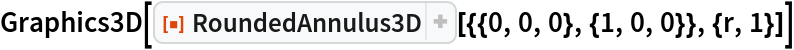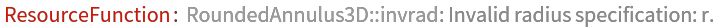Out=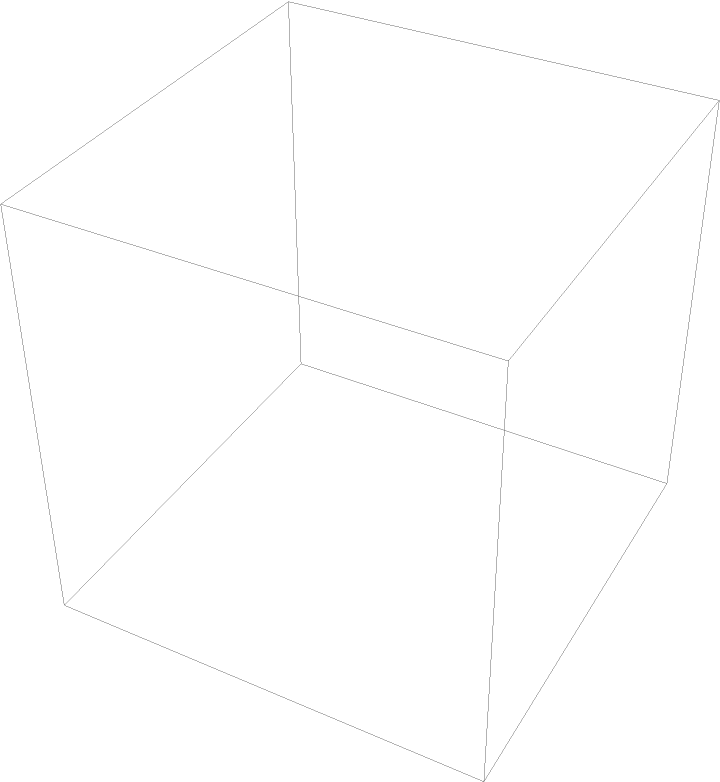When the inner radius exceeds the outer one, a warning is issued and artifacts appear:

 In:=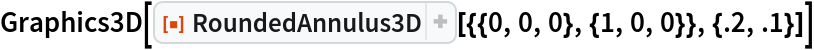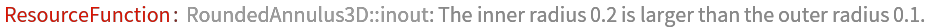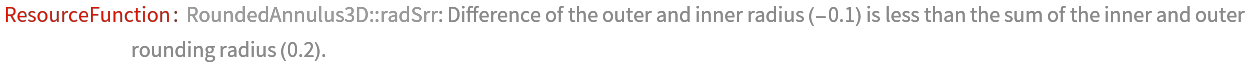Out=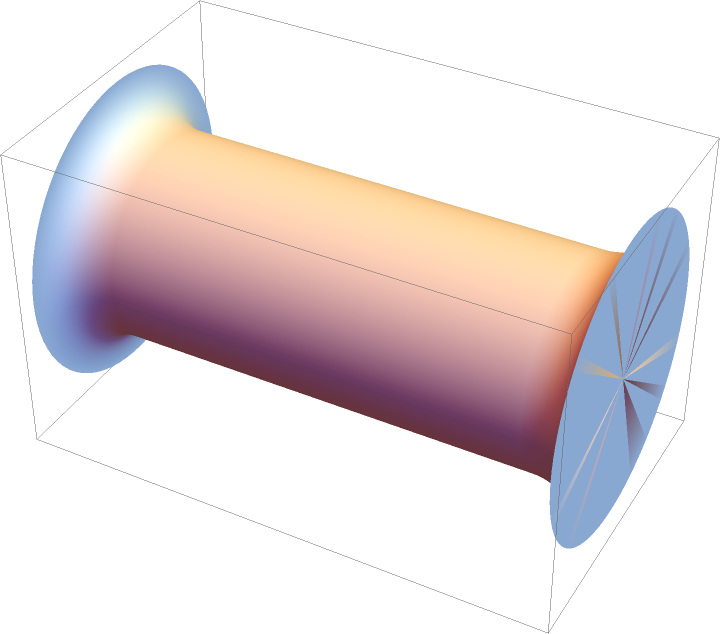When rounding radii exceed the height of the annulus, a warning is issued and artifacts appear:

 In:=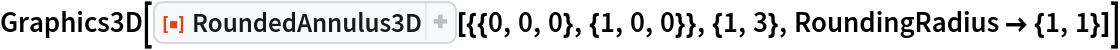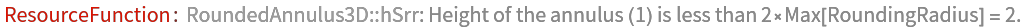Out=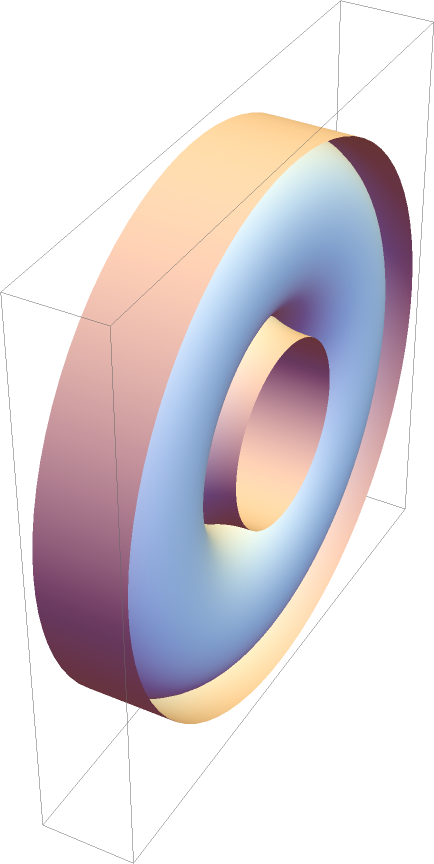When the sum of rounding radii exceeds the thickness of the annulus, a warning is issued and artifacts appear:

 In:=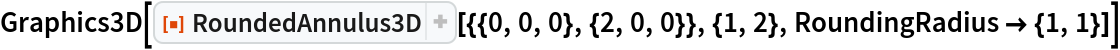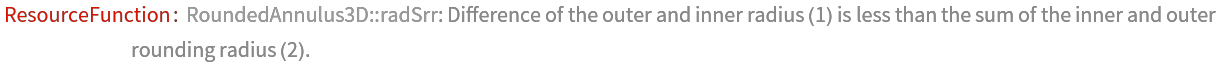Out=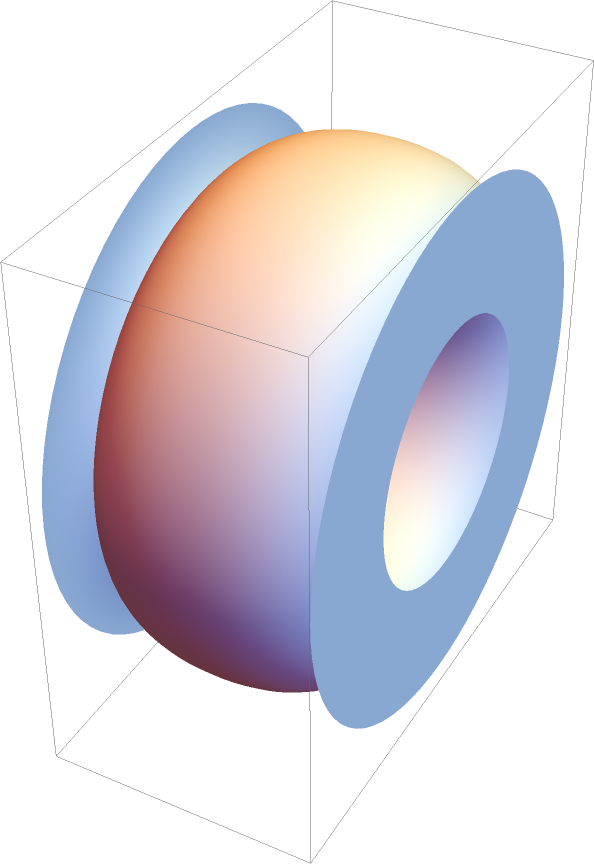### Neat Examples (4)

Random unit annuli:

 In:=Out=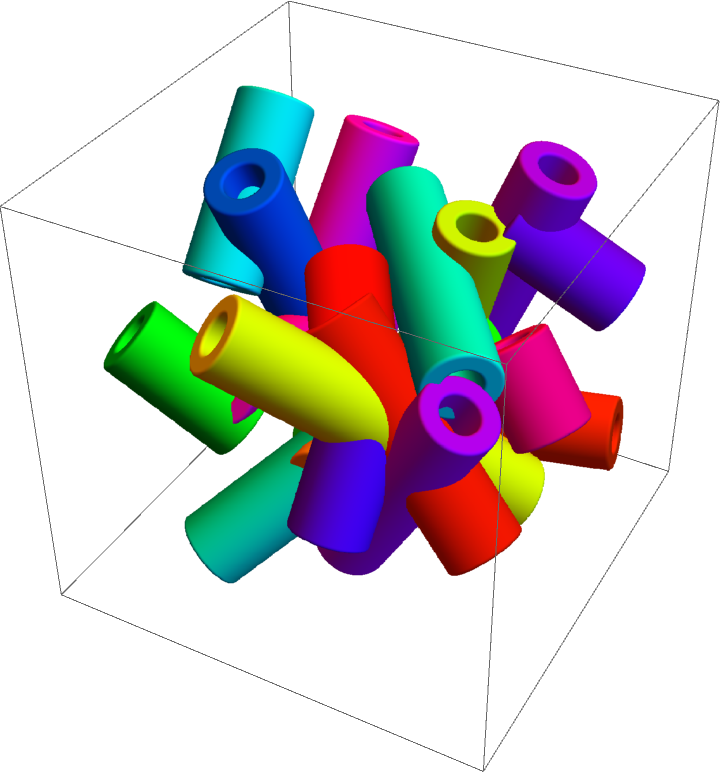Concentric annuli:

 In:=Out=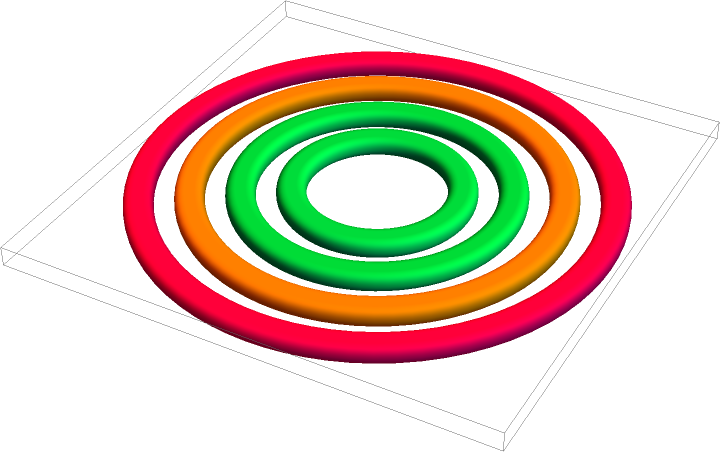Use the degenerate radius specification to create a spool-like object:

 In:=Out=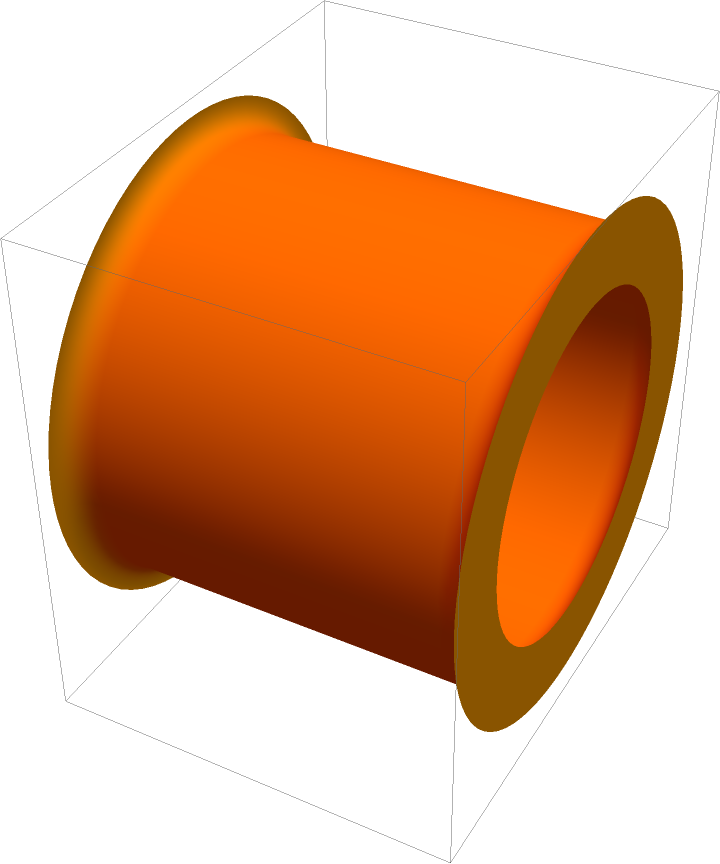Create a model of a Roman amphitheater:

 In:=Out=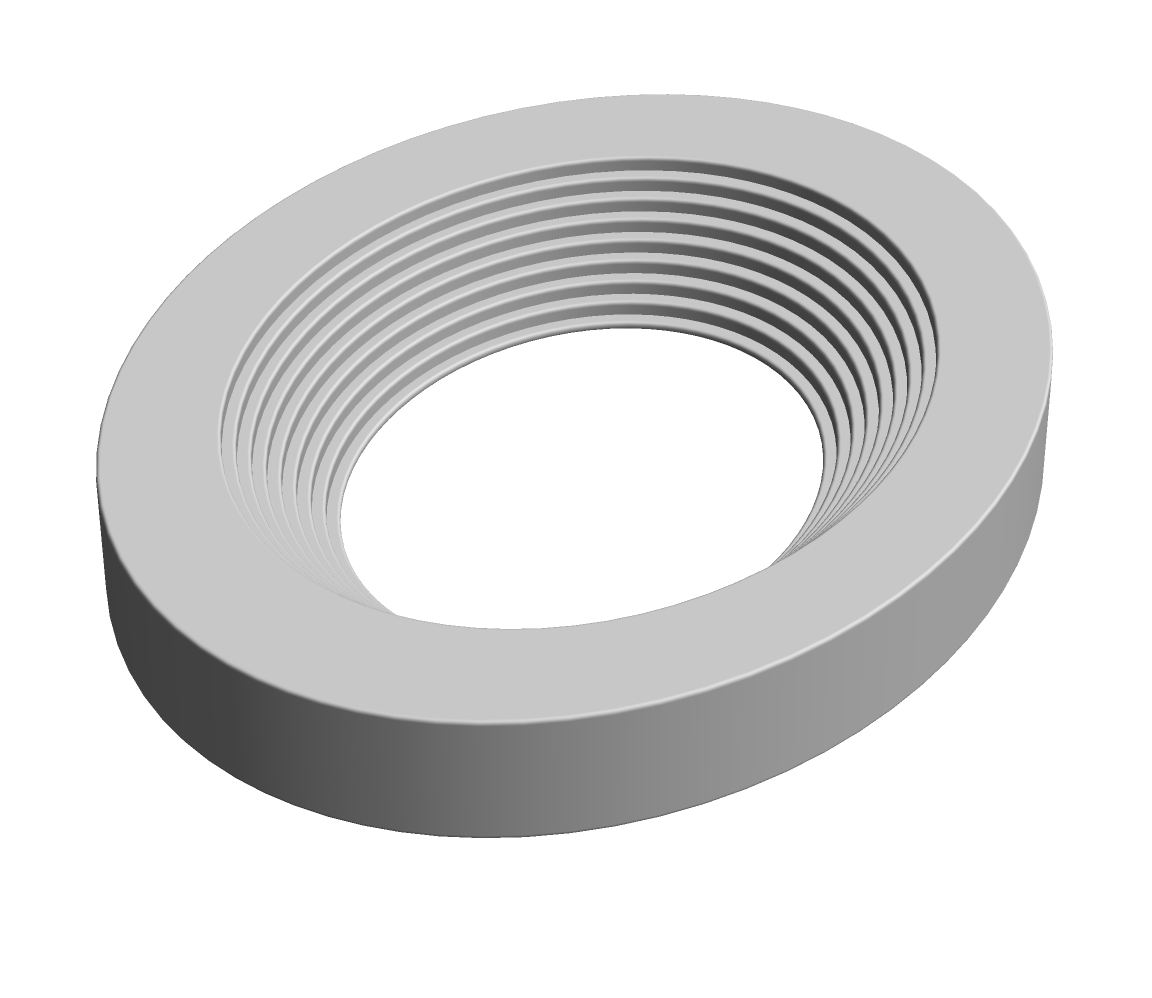Jaroslav Kysela

## Version History

• 1.0.0 – 11 January 2022

## Author Notes

Currently, no annulus sectors defined by start and end angles (akin to Annulus functionality) are implemented. The same is true for the support of Scaled.